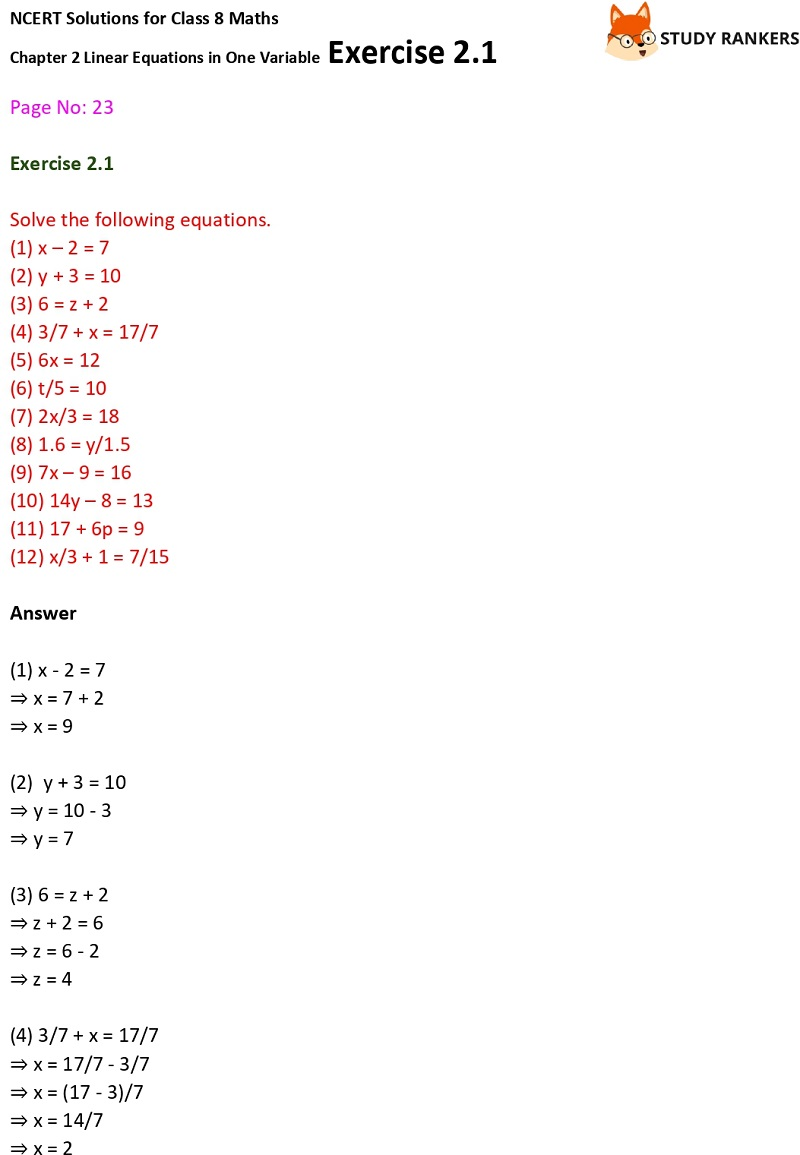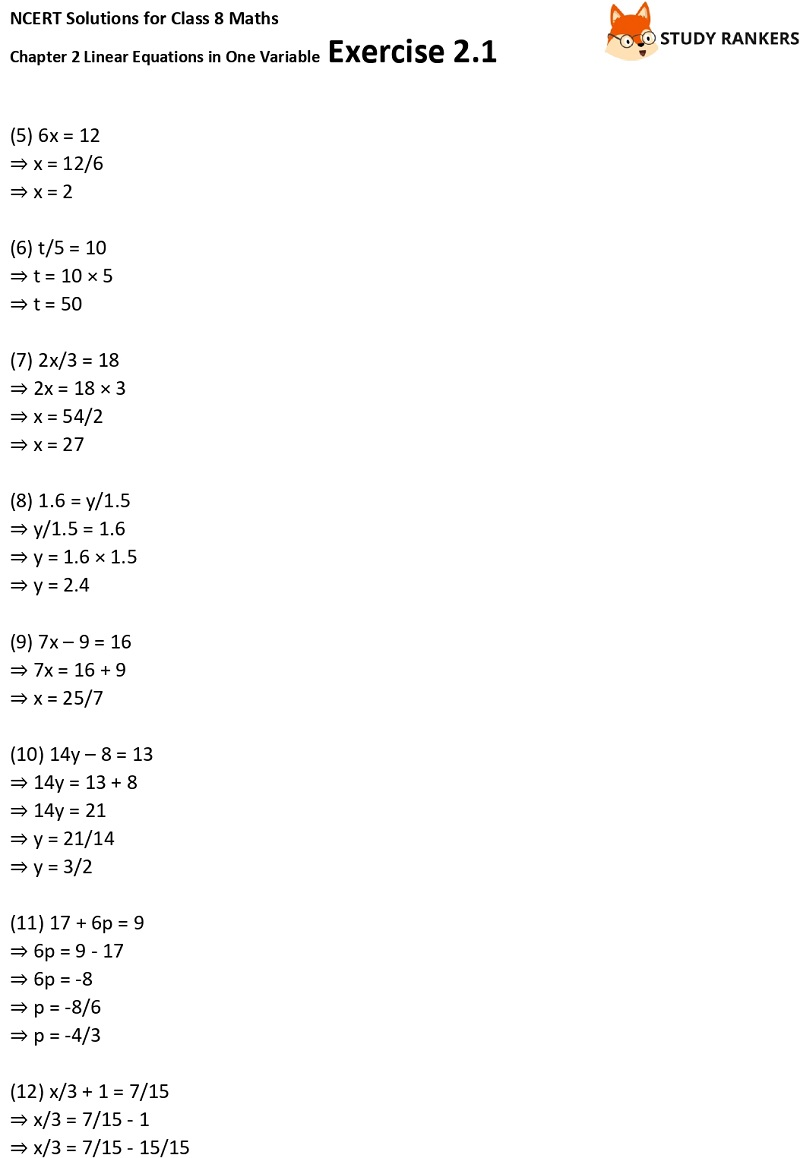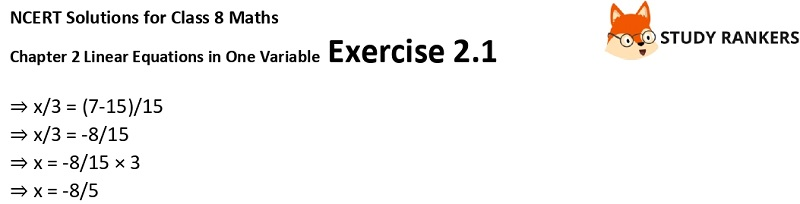>

# NCERT Solutions for Class 8 Maths Ch 2 Linear Equations in One Variable Exercise 2.1

In this page you will find Chapter 2 Linear Equations in One variable Exercise 2.1 Class 8 Maths NCERT Solutions that will help you in grasping important points inside the chapter properly. It will be useful in clearing your doubts and completing homework in no time.

You can find NCERT Solutions for Class 8 Maths which are detailed and accurate which will make student confident. In the exercise 2.1, you have to solve the given equations.X